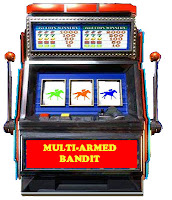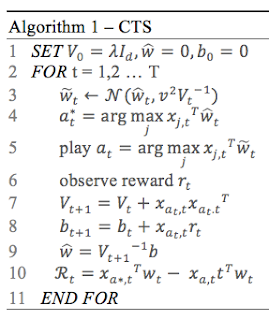## Sunday, October 20, 2019

### Contextual Thompson sampling: Theory

This post introduces the concept of multi-armed bandit (a.k.a. K-armed bandit) and the Thompson sampling for contextual bandit. The next post deals with the actual implementation of the contextual Thompson sampling in Apache Spark.

## Multi-arm Bandit problem

The multi-armed bandit problem is a well-known reinforcement learning technique,  widely used for its simplicity. Let's consider a  slot machine with n arms (bandits) with each arm having its own biased probability distribution of success. In its simplest form pulling any one of the arms gives you a  reward of either 1 for success, or 0 for failure. Our objective is to pull the arms one-by-one in sequence such that we maximize our total reward collected in the long run.Dual-arm bandit

Data scientists have developed several solutions to tackle the multi-armed bandit problem for which the 3 most common algorithms are
• Epsilon-Greedy
• Upper confidence bounds
• Thompson sampling
Epsilon-greedy
The Epsilon-greedy is the simplest algorithm to address the  exploration-exploitation trade-off.  During exploitation, the lever with highest known payout is always pulled. However, from time to time (fraction ε with  ε < 0.1) the algorithm selects a random arm to 'explore' other arms which payout is relatively unknown. The arms with known or proven highest payout are pulled (1-ε of the time)

Upper Confidence Bound (UCB)
This solution is sometimes referred as Optimism in the Face of Uncertainty principle: It assumes that the unknown mean payoffs of each arm will be as high as possible, based on historical data.

Thompson Sampling
The Thompson sampling algorithm is fundamentally a Bayesian optimization technique which core principle known as probability matching strategy can be summed as ‘play an arm according to its probability of being the best arm’.(i.e. the number of pulls for a given lever should match its actual probability of being the optimal lever)

The following diagram illustrates the difference between the upper confidence bounds and the Thompson sampling.Confidence Bounds for Multi-Armed bandit problem

## Context anyone?

The techniques described above do not make any assumption on the environment or the agent who selects the arms. There are two scenario:
• Context-free bandit: The selection of the arm with the highest rewards depends solely on the past history (prior) reward (success and failure). Such history can be modeled using a Bernoulli distribution. For instance, the probability to get a '6' playing dice does not depend on the player.
• Contextual bandit: The state of the environment (a.k.a. context) is used as an input (or model) to the selection of the arm with the highest predicted reward. The algorithm observes a new context (state), choose one action (arm), and observes an outcome (reward). In ad targeting, the selection of a banner or insert to be displayed on a web site depends on the prior rewards history associated to the demographic data of the user.
Any of the techniques applied to the multi-armed bandit can be used with and without context. The following sections focus on the contextual multi-arm bandit problem.

## Contextual Thompson sampling (CTS)

Let's dive into the key characteristics of the Thompson sampling
• We assume the prior distribution on the parameters of the distribution (unknown) of the reward for each arm.
• At each step, t, the arm is selected according to the posterior probability to be the optimal arm.
The components of the contextual Thompson sampling are
1. Model of parameters w
2. A prior distribution p(w) of the model
3. History H consisting of a context x and reward r
4. Likelihood or probability p(r|x, w) of a reward given a context x and parameters w
5. Posterior distribution computed using naïve Bayes$p(\widetilde{w}|H)=p(H|\widetilde{w}).p(\widetilde{w})/p(H))$

But how can we model a context?

Actually, a process to select the most rewarding arm is actually a predictor or a learner. Each predictor takes the context, defines as a vector of features and predicts which arm will generate the highest reward.

The predictor is a model that can be defined as
- Linear model
- Generalized linear model (i.e. logistic)
- Neural network

The algorithm is implemented as a linear model (weights w) for estimating the reward from a context x  as $w^{T}.x$
The ultimate objective for any reinforcement learning model is to extract a policy which quantifies the behavior of the agent. Given a set X of context xi and a set A of arms, the policy is defined by the selection of an arm given a context x
$\pi : X\rightarrow A$Contextual Thompson Sampling Algorithm

The sampling of the normal distribution (line 3) is described in details in the post Multivariate Normal Sampling with Scala. The algorithm computes the maximum reward estimation through sampling the normal distribution (line 4) and play the arm a* associated to it (line 5).
The parameters V and b are updated with the estimated value (line 7 and 8) and the actual reward is computed (line10) after the weights of the context are updated (line 9)

The next post describes the implementation of the contextual Thompson sampling using Scala, Nd4j and Apache Spark.